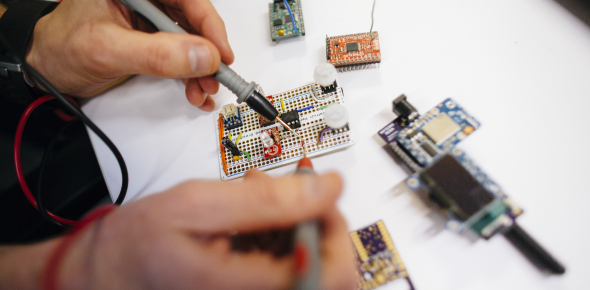# Basic Electrical Engineering Quiz: Test!

10 Questions | Attempts: 2702
ShareSettings.

• 1.
What is the unit of currency?
• A.

Ampere

• B.

Voltage

• C.

Meter

• D.

None of above

• 2.
Which of the following materials possesses the least specific resistivity?
• A.

Aluminium

• B.

Copper

• C.

Silver

• D.

Iron

• E.

None of Above

• 3.
The Curve representing Ohm's law is?
• A.

Linear

• B.

Hyperbolic

• C.

Parabolic

• D.

Triangular

• 4.
The SI unit of electrical energy is?
• A.

Watt-Second

• B.

Joule

• C.

KWh

• D.

Volt-Ampere Second

• 5.
Two wires A and B of the same Material but of different lengths L and 2L have the radius r and 2r respectively. The ratio of specific resistance will be?
• A.

1:4

• B.

1:8

• C.

1:1

• D.

1:2

• 6.
Four resistances 2Ω,4Ω,5Ω,20Ω
• A.

1Ω

• B.

2Ω

• C.

4Ω

• D.

5Ω

• 7.
Electrical conductivity of metals is typically of the order of (in Ω-1*m-1)
• A.

107

• B.

105

• C.

10-4

• D.

10-6

• 8.
Active Element in the circuit is one which
• A.

Dissipates Energy

• B.

• C.

• D.

Supplies energy

• 9.
An electric heater draws 3.5 A from a 110 V source. The resistance of the heating elements is approximately?
• A.

31Ω

• B.

3.1Ω

• C.

385Ω

• D.

38.5Ω

• 10.
We have three resistances each of 1Ω,2Ω and 3Ω. If all the three resistances are to be connected in a circuit, how many different values of equivalent resistances are possible?
• A.

Five

• B.

Six

• C.

Seven

• D.

Eight

## Related TopicsBack to top
×

Wait!
Here's an interesting quiz for you.